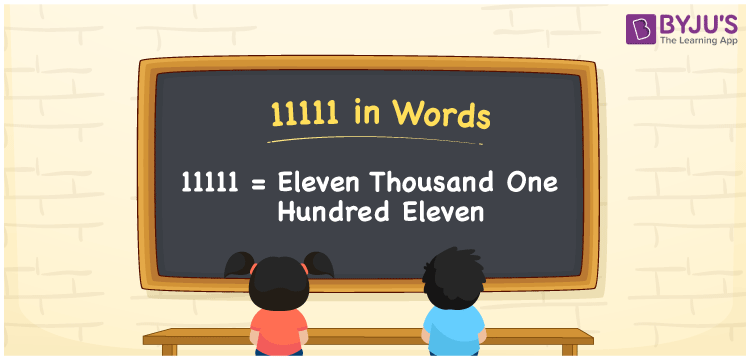# 11111 in Words

The word eleven thousand one hundred eleven is used to signify the number 11111. Using the place value of a number, the number 11111 can be quickly converted into words. As a result, a place value table must be used to write the number names. For example, if you invested Rs. 11111 on clothes, you can write “I invested Rs. Eleven thousand one hundred eleven on clothes”. This article will teach you how to write and spell the number 11111 in words.

 11111 in Words: Eleven Thousand One Hundred Eleven. Eleven Thousand One Hundred Eleven in Numerical Form: 11111.

## 11111 in English Words## How to Write 11111 in Words?

The table below shows the place value for the number 11111.

 Ten-thousands Thousands Hundreds Tens Ones 1 1 1 1 1

The following is the expanded form of the number 11111.

= 1 × Ten thousand + 1 × Thousand + 1 × Hundred + 1 × Ten + 1× One

= 1 × 10000 + 1 × 1000 + 1 × 100 + 1 × 10 + 1 × 1

= 10000 + 1000 + 100 + 10 + 1

= 11111

= Eleven thousand one hundred eleven

Hence, 11111 in words is eleven thousand one hundred eleven.

11111 in words – Eleven thousand one hundred eleven

Is 11111 an odd number? – Yes

Is 11111 an even number? – No

Is 11111 a perfect square number? – No

Is 11111 a perfect cube number? – No

Is 11111 a prime number? – No

Is 11111 a composite number? – Yes

## Frequently Asked Questions on 11111 in Words

Q1

### Write 11111 in words.

11111 in words is eleven thousand one hundred eleven.

Q2

### Simplify 11000+ 111, and express in words.

Simplifying 11000+ 111, we get 11111. Hence, 11111 in words is eleven thousand one hundred eleven.

Q3

### How to write eleven thousand one hundred eleven in numbers?

Eleven thousand one hundred eleven in numbers is 11111

Test your Knowledge on 11111 in Words